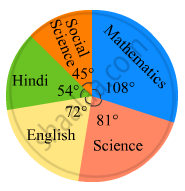Share

# In the Following Figure, the Pie-chart Shows the Marks Obtained by a Student in an Examination. If the Student Secures 440 Marks in All, Calculate His Marks in Each of the Given Subjects. - Mathematics

Course
ConceptIntroduction of Circle Graph Or Pie Chart

#### Question

In the following figure, the pie-chart shows the marks obtained by a student in an examination. If the student secures 440 marks in all, calculate his marks in each of the given subjects.#### Solution

Marks secured in mathematics = (108 x 440)/360 marks =  132 marks
Marks secured in science = (81 x 440)/360 marks = 99 marks
Marks secured in English = (72 x 440)/360  marks = 88 marks
Marks secured in Hindi = (54 x 440)/360 marks = 66 marks
Marks secured in social science = (45 x 440)/360 marks = 55 marks

Is there an error in this question or solution?

#### APPEARS IN

RD Sharma Solution for Mathematics for Class 8 by R D Sharma (2019-2020 Session) (2017 to Current)
Chapter 25: Data Handling-III (Pictorial Representation of Data as Pie Charts or Circle Graphs)
Ex. 25.2 | Q: 4 | Page no. 22

#### Video TutorialsVIEW ALL 

Solution In the Following Figure, the Pie-chart Shows the Marks Obtained by a Student in an Examination. If the Student Secures 440 Marks in All, Calculate His Marks in Each of the Given Subjects. Concept: Introduction of Circle Graph Or Pie Chart.
S